# Funding Areas

FOR MORE RESEARCH TOPICS

## The Similarities between the Nuclear and the Molecular Potential

Experimental findings show a striking similarity between the nuclear force and the force between atoms of noble gas. Figures 1 and 2 both show the potential of these forces as a function of the distance between the particles. Another similarity is the success of the nuclear liquid drop model, where a nucleus is described as an incompressible liquid drop (see , p. 139). The nuclear force is regarded as a residual force of strong interactions, while the force between noble gas atoms is a residual force of the electromagnetic force (see , pp. 100-102).

Research topic #1: Why is there a striking similarity between the nuclear force and the force between atoms of a noble gas?

Research topic #2: Does the similarities suggest something about the origin of the nuclear force?

Remark #1: The Yukawa force, whose form is (see , p. 122)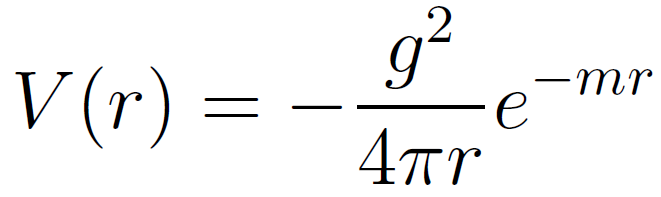cannot be regarded as an acceptable expression for the nuclear force.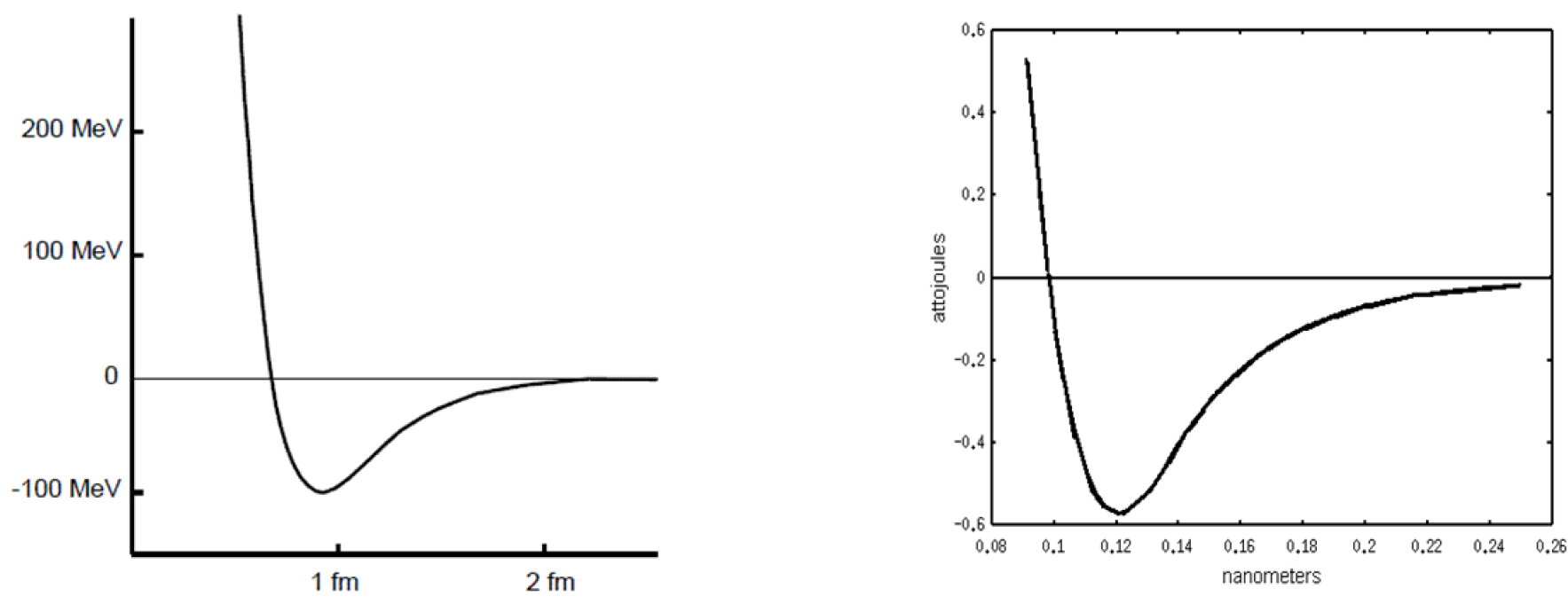Figure 1: Nuclear Force. (See , p. 97)        Figure 2: Molecular force. (See , p. 16).

Indeed, fig. 1 shows that at a short distance between nucleons, the nuclear force is strongly repulsive, whereas the Yukawa force (1) is attractive at this region.

Remark #2: In the previous decade an attempt was made to derive the nucleon-nucleon potential from QCD principles [4, 5]. However, the following arguments prove that this attempt is unacceptable. For example,  uses a pion mass of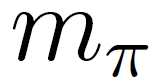=0:36 GeV, whereas the actual pion mass is below 0.14 GeV .

Another important discrepancy of  is that this article uses the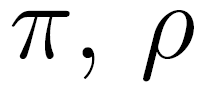mesons as interaction mediators. This is unacceptable. Indeed, an interaction mediating quantum function takes the form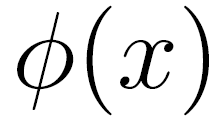, where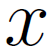denotes a single set of the four space-time coordinates. It means thatdescribes an elementary pointlike particle. For example, the textbook  (p. 300) says that this is a general attribute of presently accepted field theories: “all field theories used in current theories of elementary particles have Lagrangians of this form”. By contrast, mesons are composite states which are quark-antiquark bound systems and their function takes the form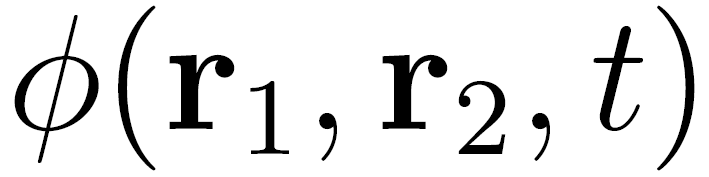.

Furthermore, the pion’s radius is about the same as that of the proton . Therefore meaning it is not a pointlike particle, which is required by an interaction mediating particle.

#### References

 S. S. M. Wong, Introductory Nuclear Physics (Wiley, New York, 1998).

 H. Haken and H. C. Wolf, Molecular Physics and Elements of Quantum Chemistry (Springer, Berlin, 2004), 2nd edition.

 M. E. Peskin and D. V. Schroeder An Introduction to Quantum Field Theory (Addison-Wesley, Reading Mass., 1995).

 N. Ishii, Aoki and T. Hatsuda, Phys. Rev. Lett., 99, 022001 (2007).

 F. Wilczek, Nature, 445, 156 (2007).

 M. Tanabashi et al. (Particle Data Group), Phys. Rev. D 98, 010001 (2018).

http://pdg.lbl.gov/2018/listings/contents_listings.html

 S.Weinberg, The Quantum Theory of Fields, Vol. I (Cambridge University Press, Cambridge, 1995). pp. 58-74.